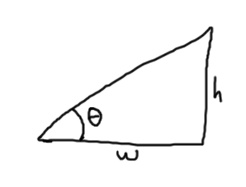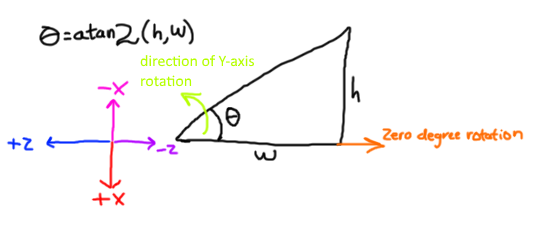# How to make head turn to Camera's lookVector direction?

I tried to use atan2 but the when the turn my character, the head will be looking at the wrong direction.

5 Likes

Code dump pls?

Also, I’m pretty sure you need to manipulate the Motor6D for the head.

Have you tried playing with the input values to atan2? Roblox uses RHR for rotations, but ends up having a LHR coordinate system when viewing top-down.

For a normal graph (i.e. +X is to the right of the paper and +Y is to the top of the paper) atan2 returns an angle of zero when given zero H and positive W:(H and W are the vertical and horizontal components of a vector)

This makes sense for a RHR coordinate system: the rotation around the up axis increases as you move in the direction your right hand’s fingers curl (i.e. counterclockwise)

Well, Roblox parts have a LHR coordinate system when looking straight down at them. To get the angle of a vector with respect to the part’s front, we need to take this into account. The front of a part (or its lookVector) is -Z axis and the left side of the part is its -X axis. The front and left sides just happen to be the best match to the coordinate system that atan2 uses to calculate angles, so what do we do?Well, we transform the inputs in a certain way to make these backwards axis into axis that atan2 can use to give you the correct angle.

See if this helps:

``````local function GetAngle(HumanoidRootPart,Camera)
local adjustedVector = HumanoidRootPart.CFrame:vectorToObjectSpace(Camera.CFrame.lookVector) -- convert the camera's lookVector into object space so that we know which way the camera is pointing relative to the part the head rotates around
local actualAngle = math.atan2(-adjustedVector.X,-adjustedVector.Z) -- this combination will turn the LHR coordinate system into a RHR angle
return actualAngle
end
``````
7 Likes

I actually answered a StackOverflow question earlier today almost exactly like this

2 Likes

You don’t even need to do that, just pivot around Neck.C0 with cameras orientation

Could you show me a working implementation of this? Because Neck.C0 is in object space whereas the Camera’s CFrame (and consequently Orientation) is in world space. The whole point of calculating the angle is to convert the world space vector into an object space angle.